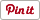\$0.99
Genres:
• Utilities
• Education

# Mathematical Formulas app review: a valuable resource for all math teachers and students

Reviews

## Introduction

Mathematical Formulas is a valuable reference application that offers common as well as complex formulas for different fields of mathematics. This is the perfect app for those who easily forget their math formulas. As a reference tool, the app makes it easier for you to solve any math problem. Developed by Antek Szadaj, the app is compatible with iPhone, iPad, and iPod touch.

The best iPhone apps for learning Math 2021

## Never Lose Your Mathematical Formulas Again

There are thousands of mathematical formulas developed over the centuries. Some of these formulas are commonly used to solve typical math problems. Unfortunately, it is beyond the usual ability of the brain to memorize all math formulas so the solution is to write down your most commonly used formulas on a piece of paper for easy reference.

The best iPad apps for learning Math

With Mathematical Formulas, you don’t have to worry about losing your formulas. The app compiles all commonly used as well as some complex formulas for easier reference. It is a valuable tool for both teachers and students of math.

The app started as a school project and was designed by a student for students. The usefulness of this app, however, goes beyond the classroom setting. It can also be used by professionals and teachers as a one-touch reference material to solve math problems.## Features of Mathematical Formulas

Mathematical Formulas offers all the basic formulas for common mathematical functions. The app covers eight general fields of mathematics, namely algebra, arithmetic, complex numbers, differentiation, geometry, integration, matrices, and trigonometry.

The best iPad apps for teachers

Each field contains dozens of basic formulas that will be useful for everyday and complex mathematics. The formulas are laid out on the screen showing exact notations with illustrations. For example, geometry formulas are clearly illustrated and show the notations for getting the measurements of volume, area, etc. Trigonometric functions, on the other hand, show graphs that illustrate the formulas.

This application offers an easy-to-use layout with a simplified workflow so you can quickly find any formula. When you open the app, you will see the different fields of mathematics on the home screen. Simply tap on a subject to get to the formula screen. From there, you can choose which formula you need. Once you are done, tap the Home button to return to the home screen.

The app has been designed for ease of use. It has a simple interface and highly responsive navigation. Take note that Mathematical Formulas is not a tutorial app. It does not offer videos or lessons. It is a reference tool which you can use to find all the math formulas you need.## Pros and Cons

Pros

• Covers eight math subjects
• Offers dozens of basic and advanced formulas
• With clear illustrations, notations, and texts
• Easy to use and responsive to touch
• Simplified workflow and friendly layout
• Works offline

Cons

• Needs to support more languages## Final Thoughts

Mathematical Formulas is a reference tool for math students and teachers. It offers a compilation of different formulas for major areas of math. It is not a tutorial app but it can be very useful when you are trying to solve complex problems.